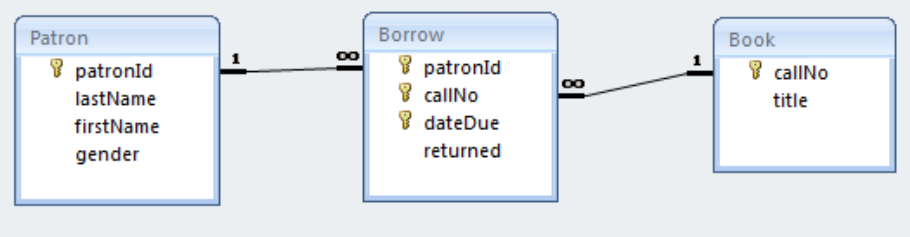# 9.2: Example

$$\newcommand{\vecs}{\overset { \rightharpoonup} {\mathbf{#1}} }$$ $$\newcommand{\vecd}{\overset{-\!-\!\rightharpoonup}{\vphantom{a}\smash {#1}}}$$$$\newcommand{\id}{\mathrm{id}}$$ $$\newcommand{\Span}{\mathrm{span}}$$ $$\newcommand{\kernel}{\mathrm{null}\,}$$ $$\newcommand{\range}{\mathrm{range}\,}$$ $$\newcommand{\RealPart}{\mathrm{Re}}$$ $$\newcommand{\ImaginaryPart}{\mathrm{Im}}$$ $$\newcommand{\Argument}{\mathrm{Arg}}$$ $$\newcommand{\norm}{\| #1 \|}$$ $$\newcommand{\inner}{\langle #1, #2 \rangle}$$ $$\newcommand{\Span}{\mathrm{span}}$$ $$\newcommand{\id}{\mathrm{id}}$$ $$\newcommand{\Span}{\mathrm{span}}$$ $$\newcommand{\kernel}{\mathrm{null}\,}$$ $$\newcommand{\range}{\mathrm{range}\,}$$ $$\newcommand{\RealPart}{\mathrm{Re}}$$ $$\newcommand{\ImaginaryPart}{\mathrm{Im}}$$ $$\newcommand{\Argument}{\mathrm{Arg}}$$ $$\newcommand{\norm}{\| #1 \|}$$ $$\newcommand{\inner}{\langle #1, #2 \rangle}$$ $$\newcommand{\Span}{\mathrm{span}}$$$$\newcommand{\AA}{\unicode[.8,0]{x212B}}$$

In this chapter we will create the tables for a Library database using DDL. Suppose we require the three tables: Book, Patron, Borrow:Figure $$\PageIndex{1}$$: Sample database to create.

The above diagram (produced from the Relationships Tool) represents the database we wish to create but where we will do so using DDL commands.

## DDL Commands

We will illustrate three DDL commands (create table, alter table, create index) as we create the library database.

 CREATE TABLE Create the Book table Create the Patron table with a primary key Create the Borrow table with a primary key and a foreign key referencing Patron ALTER TABLE Alter the Book table so it has a primary key Alter the Borrow table with a foreign key referencing Book Add an attribute named gender to Patron CREATE INDEX Create an index DROP TABLE and DROP INDEX Remove a table or index from the database

In some database environments we can run more than one command at a time; the commands would be located in a file and would be submitted as a batch to be executed. In the following we will demonstrate and run one command at a time.

## Creating the Database

Example $$\PageIndex{1}$$

Consider the following create table command which is used to create a table named Book. The table has two fields: callNo and title.

CREATE TABLE Book
(
callNo Text(50),
title Text(100)
);


The command begins with the keywords CREATE TABLE. It’s usual for keywords in DDL to be written in upper case, but it’s not required to do so. The command is just text that is parsed and executed by a command processor. If humans are expected to read the DDL then the command is typically written on several lines as shown, one part per line.

Example $$\PageIndex{2}$$

Now consider the following Create Table command which creates a table and establishes an attribute as the primary key:

CREATE TABLE Patron
(
patronId    Counter    PRIMARY KEY,
lastName    Text(50),
firstName   Text(50),
);


The primary key of Patron is the patronId field. Notice the data type is shown as Counter. After running this command you will be able to see that the Counter data type is transformed to AutoNumber.

Example $$\PageIndex{3}$$

Our last example of the create table command is one that creates a table, sets its primary key and also creates a foreign key reference to another table:

CREATE TABLE Borrow
(
patronId    Integer,
callNo      Text(50),
dateDue     DATETIME,
returned    YESNO,
PRIMARY KEY (patronId, callNo, dateDue),
FOREIGN KEY (patronId) REFERENCES Patron
);


There are several things to notice in the above command:

• The primary key is composite and so it is defined in a separate PRIMARY KEY clause.
• The data type of patron id must match the data type used in the Patron table and so the data type is defined as Integer.
• The dateDue field will hold a due date and so its data type is defined as DATETIME.
• The returned field will hold a value to indicate whether or not a book has been returned and so its data type is defined as YESNO.
• A row in the Borrow table must refer to an existing row in Patron and so we establish a relationship between Borrow and Patron using the FOREIGN KEY clause. After running this create table command you can see the relationship in Access by opening the Relationships Tool.

Example $$\PageIndex{4}$$

The Book table was created previously but there is no specification for a primary key. To add a primary key we use the alter table command as shown below.

ALTER TABLE Book


Example $$\PageIndex{5}$$

Now that Book has a primary key we can define the relationship that should exist between Borrow and Book. To do so we use the alter table command again:

ALTER TABLE Borrow
REFERENCES Book (callNo);


Example $$\PageIndex{6}$$

Notice that the Patron table does not have a gender attribute. To add this later on we can use the alter table command:

ALTER TABLE Patron
COLUMN gender Text(6);


Example $$\PageIndex{7}$$

For performance reasons we can add indexes to a table. DDL provides create index and drop index commands for managing these structures. To create an index for Patron on the combination last name and first name, we can execute:

CREATE INDEX PatronNameIndex
ON Patron (LastName, FirstName);


Example $$\PageIndex{8}$$

To remove the above index we need to identify the index by name:

DROP INDEX PatronNameIndex;


Example $$\PageIndex{9}$$

To remove a table we use the drop table command.

DROP TABLE Person;


## Exercises

Exercise $$\PageIndex{1}$$

Try running the commands in examples 1 through 3. After running a DDL statement open the corresponding table in Design View and verify that the statement worked as intended.

Exercise $$\PageIndex{2}$$

Try running the commands in examples 4 through 6. After running a DDL statement open the corresponding table in Design View and verify that the statement worked as intended.

Exercise $$\PageIndex{3}$$

The effect of executing the commands in the first 6 examples can be accomplished by 3 create table commands. Example 9 shows a drop table command; use similar drop commands to delete all the tables you created in exercises 1 and 2. Now, write 3 create table commands that have the same effect as examples 1 through 6. After running the DDL statements open the relationships tool to verify your commands created the 3 tables and the 2 relationships.

Exercise $$\PageIndex{4}$$

Example 7 creates an index. Run this command in your database and then verify the index has been created. You can view index information by clicking the Indexes icon:

Notice that the (primary) index has a name that was generated by MS Access.

Exercise $$\PageIndex{5}$$

Consider an ERD from the previous chapter. Write the DDL that could create the required relations.